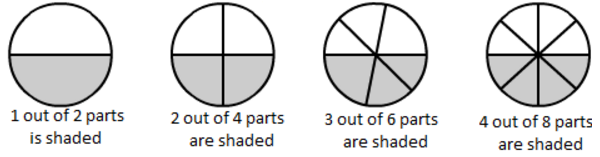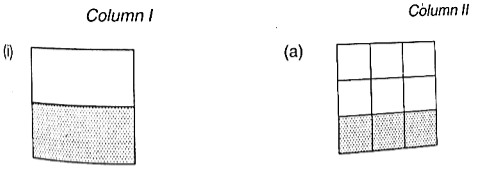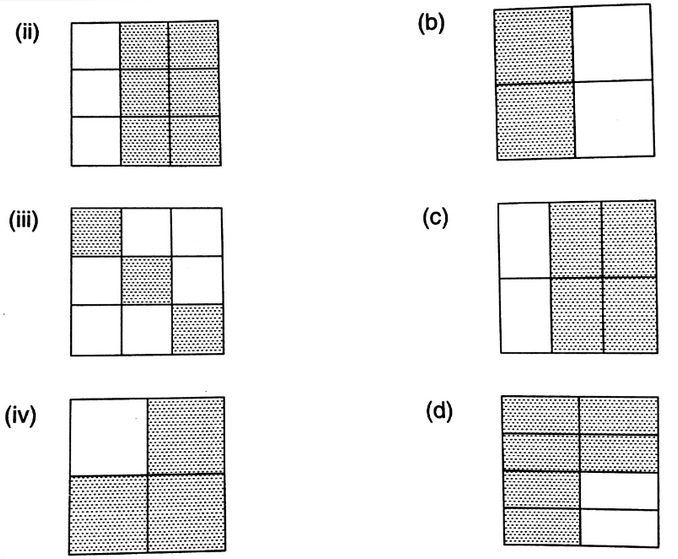×#### Thank you for registering.

One of our academic counsellors will contact you within 1 working day.

Click to Chat

1800-1023-196

+91-120-4616500

CART 0

• 0

MY CART (5)

Use Coupon: CART20 and get 20% off on all online Study Material

ITEM
DETAILS
MRP
DISCOUNT
FINAL PRICE
Total Price: Rs.

There are no items in this cart.
Continue Shopping• Complete JEE Main/Advanced Course and Test Series
• OFFERED PRICE: Rs. 15,900
• View Details

```Chapter 6: Fractions – Exercise 6.5

Question: 1

(i)(ii)Solution:

(i)

Fraction = 1/2

Fraction = 2/4 = 1/2

Fraction = 3/6 = 1/2

Fraction = 4/8 = 1/2

Yes, they are equivalent

(ii)

Fraction = 5/15 = 1/3

Fraction = 3/9 = 1/3

Fraction = 2/6 = 1/3

Fraction = 1/3

Yes, they are equivalent

Question: 2

Write the fractions and match fractions in column I with the equivalent fractions in column II.Solution:

(i) (b)

(ii) (c)

(iii) (a)

(iv) (d)

Question: 3

Replace * in each of the following by the correct number:

(i) 2/7 = 6/*

(ii) 5/8 = 10/*

(iii) 4/5 = */20

(iv) 45/60 = 15/*

(v) 18/24 = */4

Solution:

(i) 2/7 = 6/21

(ii) 5/8 = 10/16

(iii) 4/5 = 16/20

(iv) 45/60 = 15/20

(v) 18/24 = 3/8

Question: 4

Find the equivalent fraction of 35, having:

(i) Numerator 9

(ii) Denominator 30

(iii) Denominator 21

(iv) Numerator 40

Solution:

(i)  3/5 = 9

Consider the numerator = 9

As 3 × 3 = 9, we will multiply both the numerator and denominator by 3, we have

3/5 × 3/3 = 9/15

(ii)  3/5 = 30

Consider the denominator = 30

As 5 × 6 = 30, we multiply both the numerator and denominator by 6, we have

3/5 × 6/6 = 18/30 = 3/5

(iii) 3/5 = 21

Consider the denominator = 21

As 3 × 7 = 21, we multiply both the numerator and denominator by 7, we have

3/5 × 7/7 = 21/35

(iv) 3/5 = 40

Consider the numerator = 40

As 5 × 8 = 40, we multiply both the numerator and denominator by 8, we have

3/5 × 8/8 = 24/40

Question: 5

Find the fraction equivalent to 45/60, having:

(i) Numerator 15

(ii) Denominator 4

(iii) Denominator 240

(iv) Numerator 135

Solution:

(i) 45/60 = 15

Consider the numerator = 15

As 45 ÷ 3 = 15, we will divide both the numerator and denominator by 3, we have,

45/60 ÷ 3/3 = 15/20

(ii) 45/60 = 4

Consider the denominator = 4

As 60 ÷ 15 = 4, We divide both the numerator and denominator by 15,

we have ,

45/60 ÷ 15/15 = 3/4

(iii) 45/60 = 240

Consider the denominator = 240

As 60 × 4 = 240, we multiply both the numerator and denominator by 4, we have

45/60 × 4/4 = 180/240

(iv) 45/60 = 135

Consider the numerator = 135

As 45 x 3 = 135, we multiply both the numerator and denominator by 3, we have

45/60 × 3/3 = 135/180

Question: 6

Find the fraction equivalent to 35/42, having:

(i) Numerator 15

(ii) Denominator 18

(iii) Denominator 30

(iv) Numerator 30

Solution:

Firstly, we will reduce 35/42 into the lowest term. Now, we will divide both the numerator and denominator by the HCFs of 35 and 42, i.e 7, we have

35/42 ÷ 7/7 = 5/6

(i) 5/6 = 15

Consider the numerator = 15

As 5 × 3 = 15, we will multiply both the numerator and denominator by 3, we have

5/6 × 3/3 = 15/18

(ii) 5/6 = 18

Consider the denominator = 18

As 6 × 3 = 18, we multiply both the numerator and denominator by 3, we have

5/6 × 3/3 = 15/18

(iii) 5/6 = 30

Consider the denominator = 30

As 6 × 5 = 30, we multiply both the numerator and denominator by 5, we have

5/6 × 5/5 = 25/30

(iv) 5/6 = 30

Consider the numerator = 30

As 5 × 6 = 30, we multiply both the numerator and denominator by 6, we have

5/6 × 6/6 = 30/36

Question: 7

Check whether the given fractions are equivalent:

(i)  5/9, 30/54

(ii)  2/7, 16/42

(iii)  7/13, 5/11

(iv)  4/11, 32/88

(v)  3/10, 12/50

(vi)  9/27, 25/75

Solution:

(i) 5/9 × 6/6 = 30/54

Hence, the given fractions are equivalent

(ii) 2/7 × 8/8 = 16/42

27 is not equivalent to 16/42

(iii) 7/13 × 5/5 = 35/65

5/11 × 7/7 = 35/77

7/13 is not equivalent to 5/11

(iv) 4/11 × 8/8 = 32/88

4/11 is equivalent to 32/88

(v) 3/10 × 4/4 = 12/50

3/10 is not equivalent 12/50

(vi) 9/27 × 13/13 = 25/75

9/27 is equivalent 25/75

Question: 8

Match the equivalent fractions and write another 2 for each:

(i)  250/400
(a)  2/3

(ii)  180/200
(b)  2/5

(iii)  660/990
(c)  1/2

(iv)  180/360
(d)  5/8

(v)  220/550
(e)  9/10

Solution:

The correct matches for the above question are given below:

(i) (d),  250/400, 5/8

(ii) (e),  180/200, 9/10

(iii) (a),  660/990, 2/3

(iv) (c),  180/360, 1/2

(v) (b),  220/550, 2/5

Question: 9

Write some equivalent fractions which contain all digits from 1 to 9 once only.

Solution:

2/6 = 3/9 = 58/174, 2/4 = 3/6 = 79/158

Question: 10

Ravish had 20 pencils , sikha had 50 pencils and priya had 80 pencils. After 4 months, Ravish used up 10 pencils, sikha used up 25 pencils and priya used 40 pencils. What fraction did each use up? Check if each has used up an equal fraction of their pencils?

Solution:

Total pencils Ravish had = 20

Pencils used by Ravish = 10

Fraction of pencils used by ravish = 10 ÷ 10/20 ÷ 10 = 12 (Dividing both the numerator &

denominator by the HCFs of 10 & 20 ) Total pencils Shikha had = 50 Pencils used by

Shikha = 25 Fraction of pencils used by Shikha = 25 ÷ 25/50 ÷ 25 = 12 (Dividing both the numerator & denominator by the HCFs of 25 & 50)

Total pencils Priya had = 80

Pencils used by Priya = 40

Fraction of pencils used by Priya = 40 ÷ 40/80 ÷ 40 = 12 (Dividing both the numerator

& denominator by the HCFs of 40 & 80)

Yes, each of them has utilized an equal fraction of pencils.
```### Course Features

• 728 Video Lectures
• Revision Notes
• Previous Year Papers
• Mind Map
• Study Planner
• NCERT Solutions
• Discussion Forum
• Test paper with Video Solution